``````library(ggplot2)
library(ComplexUpset)``````

## Prepare the datasets

``````movies = as.data.frame(ggplot2movies::movies)
head(movies, 3)``````
A data.frame: 3 × 24
title year length budget rating votes r1 r2 r3 r4 r9 r10 mpaa Action Animation Comedy Drama Documentary Romance Short
<chr> <int> <int> <int> <dbl> <int> <dbl> <dbl> <dbl> <dbl> <dbl> <dbl> <chr> <int> <int> <int> <int> <int> <int> <int>
1 \$ 1971 121 NA 6.4 348 4.5 4.5 4.5 4.5 4.5 4.5 0 0 1 1 0 0 0
2 \$1000 a Touchdown 1939 71 NA 6.0 20 0.0 14.5 4.5 24.5 4.5 14.5 0 0 1 0 0 0 0
3 \$21 a Day Once a Month 1941 7 NA 8.2 5 0.0 0.0 0.0 0.0 24.5 24.5 0 1 0 0 0 0 1
``````genres = colnames(movies)[18:24]
genres``````
1. ‘Action’
2. ‘Animation’
3. ‘Comedy’
4. ‘Drama’
5. ‘Documentary’
6. ‘Romance’
7. ‘Short’

Convert the genre indicator columns to use boolean values:

``````movies[genres] = movies[genres] == 1
t(head(movies[genres], 3))``````
A matrix: 7 × 3 of type lgl
1 2 3
Action FALSE FALSE FALSE
Animation FALSE FALSE TRUE
Comedy TRUE TRUE FALSE
Drama TRUE FALSE FALSE
Documentary FALSE FALSE FALSE
Romance FALSE FALSE FALSE
Short FALSE FALSE TRUE

To keep the examples fast to compile we will operate on a subset of the movies with complete data:

``````movies[movies\$mpaa == '', 'mpaa'] = NA
movies = na.omit(movies)``````

Utility for changing output parameters in Jupyter notebooks (IRKernel kernel), not relevant if using RStudio or scripting R from terminal:

``````set_size = function(w, h, factor=1.5) {
s = 1 * factor
options(
repr.plot.width=w * s,
repr.plot.height=h * s,
repr.plot.res=100 / factor,
jupyter.plot_mimetypes='image/png',
jupyter.plot_scale=1
)
}``````

## 0. Basic usage

There are two required arguments:

• the first argument is expected to be a dataframe with both group indicator variables and covariates,
• the second argument specifies a list with names of column which indicate the group membership.

Additional arguments can be provided, such as `name` (specifies `xlab()` for intersection matrix) or `width_ratio` (specifies how much space should be occupied by the set size panel). Other such arguments are discussed at length later in this document.

``````set_size(8, 3)
upset(movies, genres, name='genre', width_ratio=0.1)``````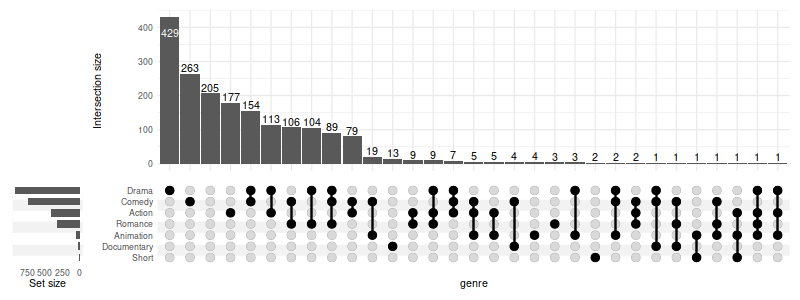### 0.1 Selecting intersections

We will focus on the intersections with at least ten members `(min_size=10)` and on a few variables which are significantly different between the intersections (see 2. Running statistical tests).

When using `min_size`, the empty groups will be skipped by default (e.g. Short movies would have no overlap with size of 10). To keep all groups pass `keep_empty_groups=TRUE`:

``````set_size(8, 3)
(
upset(movies, genres, name='genre', width_ratio=0.1, min_size=10, wrap=TRUE, set_sizes=FALSE)
+ ggtitle('Without empty groups (Short dropped)')
+    # adding plots is possible thanks to patchwork
upset(movies, genres, name='genre', width_ratio=0.1, min_size=10, keep_empty_groups=TRUE, wrap=TRUE, set_sizes=FALSE)
+ ggtitle('With empty groups')
)``````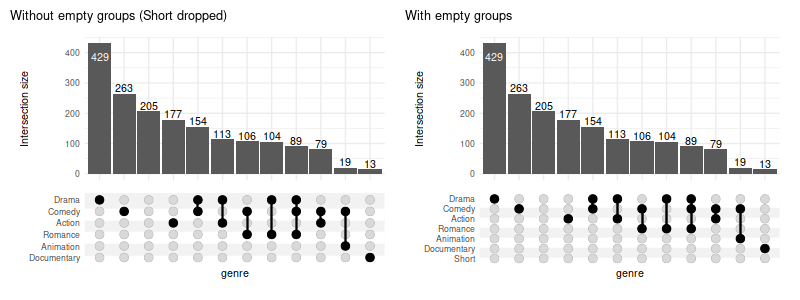When empty columns are detected a warning will be issued. The silence it, pass `warn_when_dropping_groups=FALSE`. Complimentary `max_size` can be used in tandem.

You can also select intersections by degree (`min_degree` and `max_degree`):

``````set_size(8, 3)
upset(
movies, genres, width_ratio=0.1,
min_degree=3,
)``````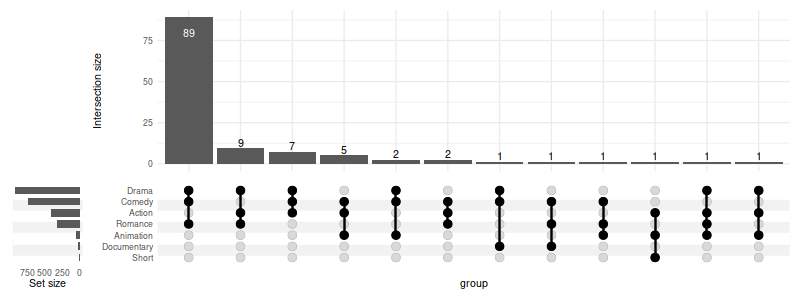Or request a constant number of intersections with `n_intersections`:

``````set_size(8, 3)
upset(
movies, genres, width_ratio=0.1,
n_intersections=15
)``````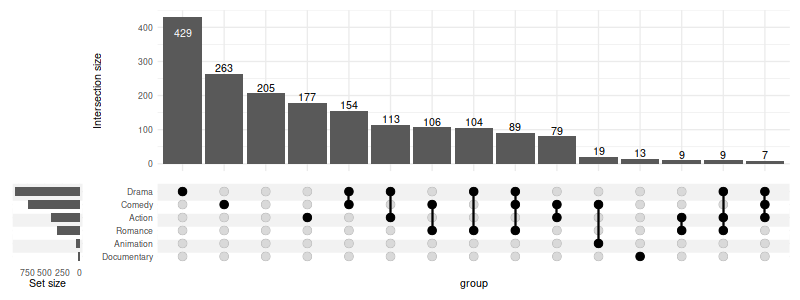### 0.2 Region selection modes

There are four modes defining the regions of interest on corresponding Venn diagram:

• `exclusive_intersection` region: intersection elements that belong to the sets defining the intersection but not to any other set (alias: distinct), default
• `inclusive_intersection` region: intersection elements that belong to the sets defining the intersection including overlaps with other sets (alias: intersect)
• `exclusive_union` region: union elements that belong to the sets defining the union, excluding those overlapping with any other set
• `inclusive_union` region: union elements that belong to the sets defining the union, including those overlapping with any other set (alias: union)

Example: given three sets \(A\), \(B\) and \(C\) with number of elements defined by the Venn diagram below

``````abc_data = create_upset_abc_example()

abc_venn = (
ggplot(arrange_venn(abc_data))
+ coord_fixed()
+ theme_void()
+ scale_color_venn_mix(abc_data)
)

(
abc_venn
+ geom_venn_region(data=abc_data, alpha=0.05)
+ geom_point(aes(x=x, y=y, color=region), size=1)
+ geom_venn_circle(abc_data)
+ geom_venn_label_set(abc_data, aes(label=region))
+ geom_venn_label_region(
abc_data, aes(label=size),
outwards_adjust=1.75,
position=position_nudge(y=0.2)
)
+ scale_fill_venn_mix(abc_data, guide=FALSE)
)``````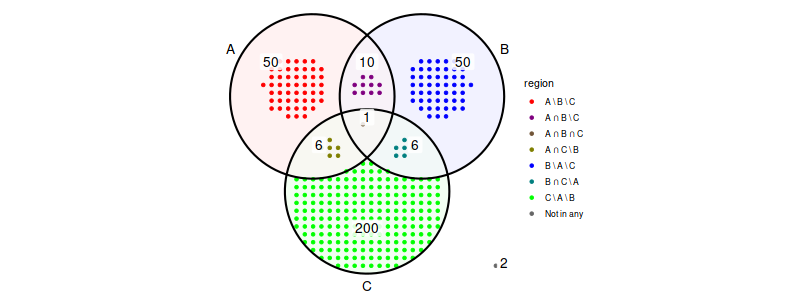For the above sets \(A\) and \(B\) the region selection modes correspond to region of Venn diagram defined as follows:

• exclusive intersection: \((A \cap B) \setminus C\)
• inclusive intersection: \(A \cap B\)
• exclusive union: \((A \cup B) \setminus C\)
• inclusive union: \(A \cup B\)

and have the total number of elements as in the table below:

members  mode exclusive int. inclusive int. exclusive union inclusive union
(A, B) 10 11 110 123
(A, C) == (B, C) 6 7 256 273
(A) == (B) 50 67 50 67
(C) 200 213 200 213
(A, B, C) 1 1 323 323
() 2 2 2 2
``````set_size(6, 6.5)
simple_venn = (
abc_venn
+ geom_venn_region(data=abc_data, alpha=0.3)
+ geom_point(aes(x=x, y=y), size=0.75, alpha=0.3)
+ geom_venn_circle(abc_data)
+ geom_venn_label_set(abc_data, aes(label=region), outwards_adjust=2.55)
)
highlight = function(regions) scale_fill_venn_mix(
abc_data, guide=FALSE, highlight=regions, inactive_color='NA'
)

(
(
simple_venn + highlight(c('A-B')) + labs(title='Exclusive intersection of A and B')
| simple_venn + highlight(c('A-B', 'A-B-C')) + labs(title='Inclusive intersection of A and B')
) /
(
simple_venn + highlight(c('A-B', 'A', 'B')) + labs(title='Exclusive union of A and B')
| simple_venn + highlight(c('A-B', 'A-B-C', 'A', 'B', 'A-C', 'B-C')) + labs(title='Inclusive union of A and B')
)
)``````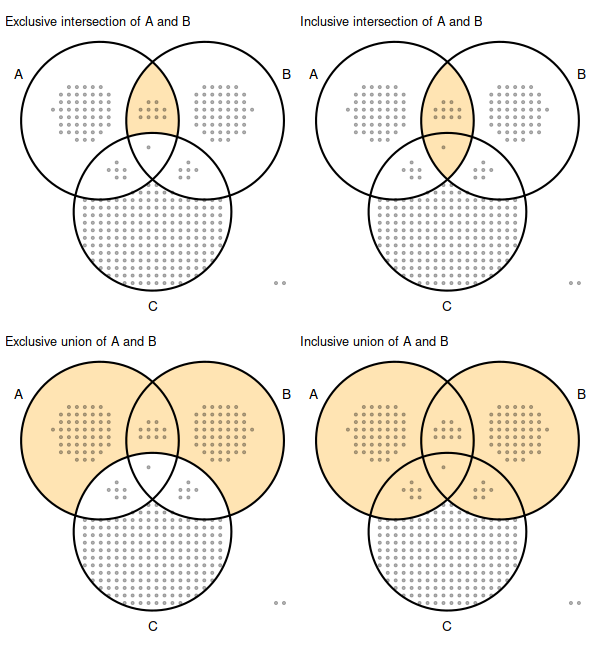When customizing the `intersection_size()` it is important to adjust the mode accordingly, as it defaults to `exclusive_intersection` and cannot be automatically deduced when user customizations are being applied:

``````set_size(8, 4.5)
abc_upset = function(mode) upset(
abc_data, c('A', 'B', 'C'), mode=mode, set_sizes=FALSE,
encode_sets=FALSE,
queries=list(upset_query(intersect=c('A', 'B'), color='orange')),
base_annotations=list(
'Size'=(
intersection_size(
mode=mode,
mapping=aes(fill=exclusive_intersection),
size=0,
text=list(check_overlap=TRUE)
) + scale_fill_venn_mix(
data=abc_data,
guide=FALSE,
colors=c('A'='red', 'B'='blue', 'C'='green3')
)
)
)
)

(
(abc_upset('exclusive_intersection') | abc_upset('inclusive_intersection'))
/
(abc_upset('exclusive_union') | abc_upset('inclusive_union'))
)``````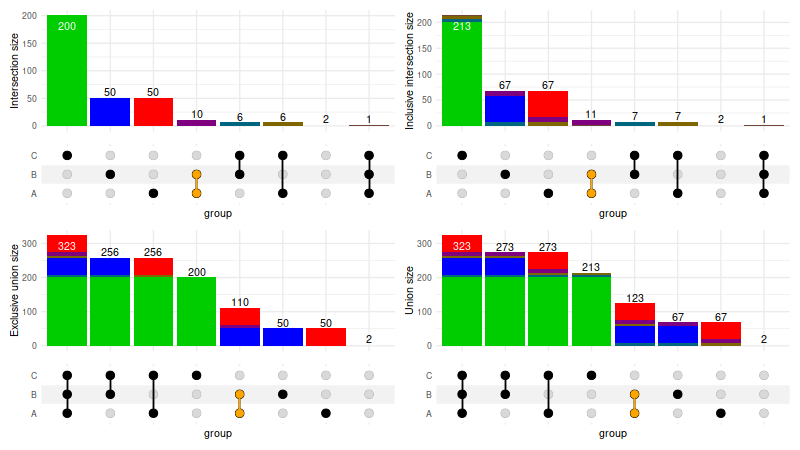### 0.3 Displaying all intersections

To display all possible intersections (rather than only the observed ones) use `intersections='all'`.

Note 1: it is usually desired to filter all the possible intersections down with `max_degree` and/or `min_degree` to avoid generating all combinations as those can easily use up all available RAM memory when dealing with multiple sets (e.g. all human genes) due to sheer number of possible combinations

Note 2: using `intersections='all'` is only reasonable for mode different from the default exclusive intersection.

``````set_size(8, 3)
upset(
movies, genres,
width_ratio=0.1,
min_size=10,
mode='inclusive_union',
base_annotations=list('Size'=(intersection_size(counts=FALSE, mode='inclusive_union'))),
intersections='all',
max_degree=3
)``````